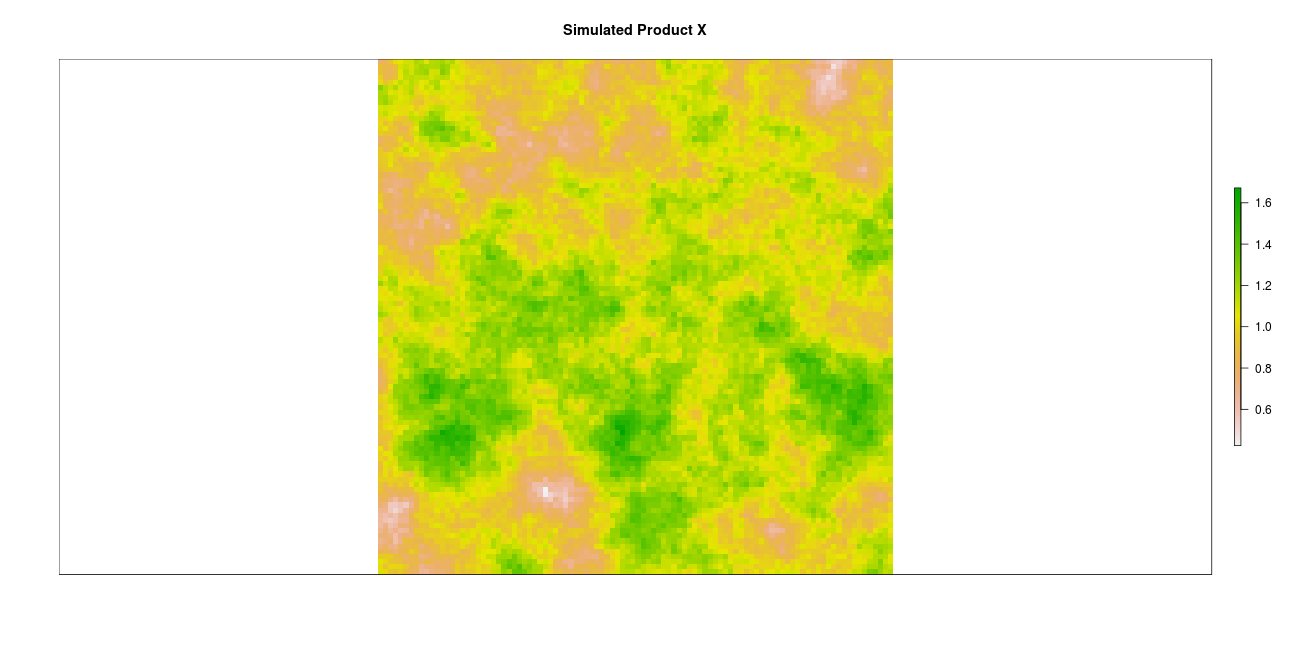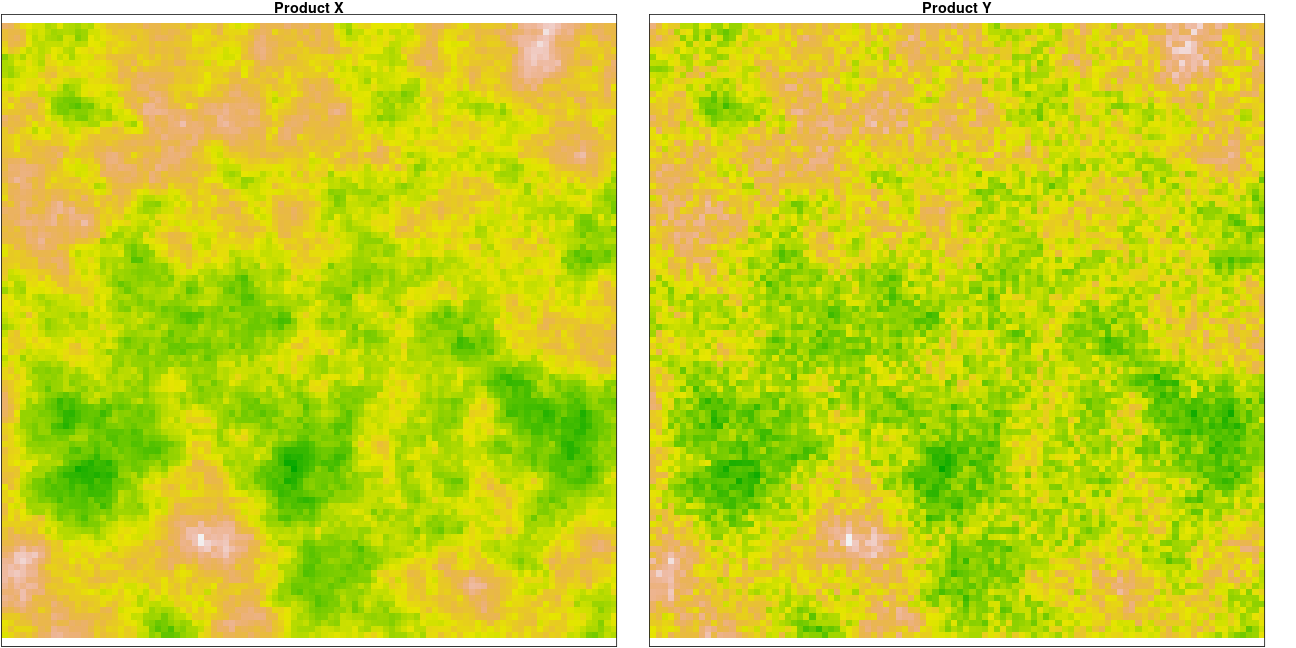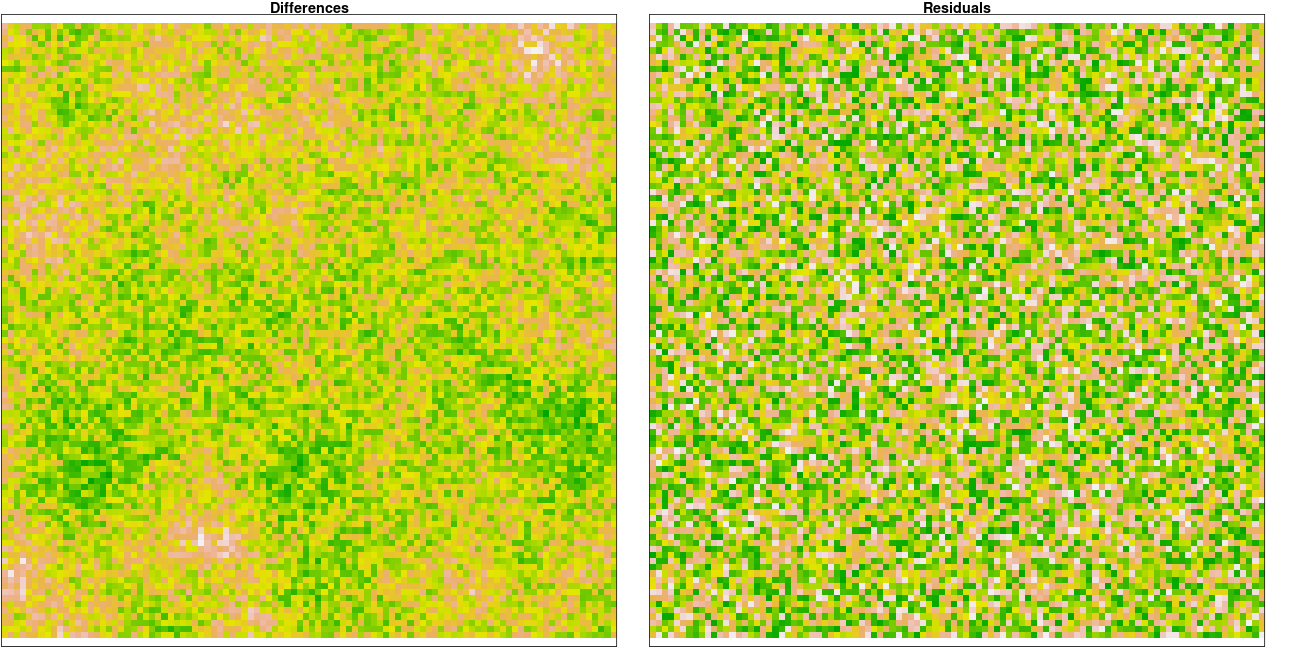# Goal

The evaluation of a satellite product normally includes exploring its consistency with respect to former products. For this comparison to be considered satisfactory, it should be possible to describe the given relationship by an spatially-independent simple model, best a linear model. Spatial independence implies that the same model links both products in different geographic regions, and the statistical method to proof it involves exploring the spatial distribution of the residuals and testing for spatial autocorrelation.
Here I present an example with artificial data. As we control the way we generate them, artificial data allows us to verify that our conclusions are actually correct.

knitr::opts_chunk\$set(results='hide', echo=FALSE, warning=FALSE, message=FALSE,
fig.path = "figure/diffsppattern_")

# 1. The Spatial distribution of differences and residuals

Let’s call our former and new products X and Y respectively, and let’s investigate whether a linear relationship between them exisits:
Y = a + bX + e
We must estimate the parameters and explore the spatial structure of e. Note that the spatial distribution of the residuals e will be different from that of Y-X unless the slope b is equal to 1. We highlight the difference in this section.

## 1.1 Create artificial data

### 1.1.1 Create artificial product

We simulate the product as a random field with autocorrelation defined by an exponential variogram.### 1.1.2 Create another product as linear transform

We simulate a newer product Y as a linear function of X plus some random noise.

y <- 0.25 + x*1.5
e <- y*0
e[] <- runif(10000,-0.2,0.2)
y <- y + e## 1.2 Estimate the model

Now we pretend we do not know the parameters and estimate them through OLS

lmod <- lm(y[] ~ x[])
summary(lmod)
##
## Call:
## lm(formula = y[] ~ x[])
##
## Residuals:
##       Min        1Q    Median        3Q       Max
## -0.201690 -0.099796 -0.000085  0.099323  0.202980
##
## Coefficients:
##             Estimate Std. Error t value Pr(>|t|)
## (Intercept) 0.240168   0.007264   33.06   <2e-16 ***
## x[]         1.509237   0.006710  224.91   <2e-16 ***
## ---
## Signif. codes:  0 '***' 0.001 '**' 0.01 '*' 0.05 '.' 0.1 ' ' 1
##
## Residual standard error: 0.1152 on 9998 degrees of freedom
## Multiple R-squared:  0.835,  Adjusted R-squared:  0.835
## F-statistic: 5.058e+04 on 1 and 9998 DF,  p-value: < 2.2e-16

As expected, we retrieve values very close to those of a and b that we had set.

## 1.3 Compare Spatial Distribution of Differences and Residuals

We first plot the fields of Differences and Residuals.Note that some spatial autocorrelation can be observed in the raster of differences that is not present in the residuals.
In order to actually measure spatial autocorrelation we use the Moran index, which is close to 0 for spatial randomness, higher for aggregation and negative for overdispersion. The significance of the Moran index can be tested analytically or by permutation simulations (which can cope with irregular boundaries)

Moran test (analytic form)

##             Moran I statistic Expectation p.value
## Residuals              -0.007           0   0.906
## Differences             0.325           0   0.000

Moran test (permutation method)

##             Moran I statistic Expectation p.value
## Residuals              -0.007       0.000   0.896
## Differences             0.325       0.002   0.005

Both methods indicate that the value of the Moran index is 0 for the residuals, thus the null hypothesis of spatial randomness cannot be rejected (p-value>>0.05). In contrast, the Moran index for the differences is significantly higher than 0 (p-value << 0.05), indicating spatial aggregation.

The spatial pattern of the differences between two linearly related spatial variables can show aggregation even in the case of spatial independence, unless the slope of the relationship is 1. Comparing the spatial distribution of the difference Y-X is misleading. The spatial independence of the relationship must be investigated through the analysis of the spatial distribution of the residuals.